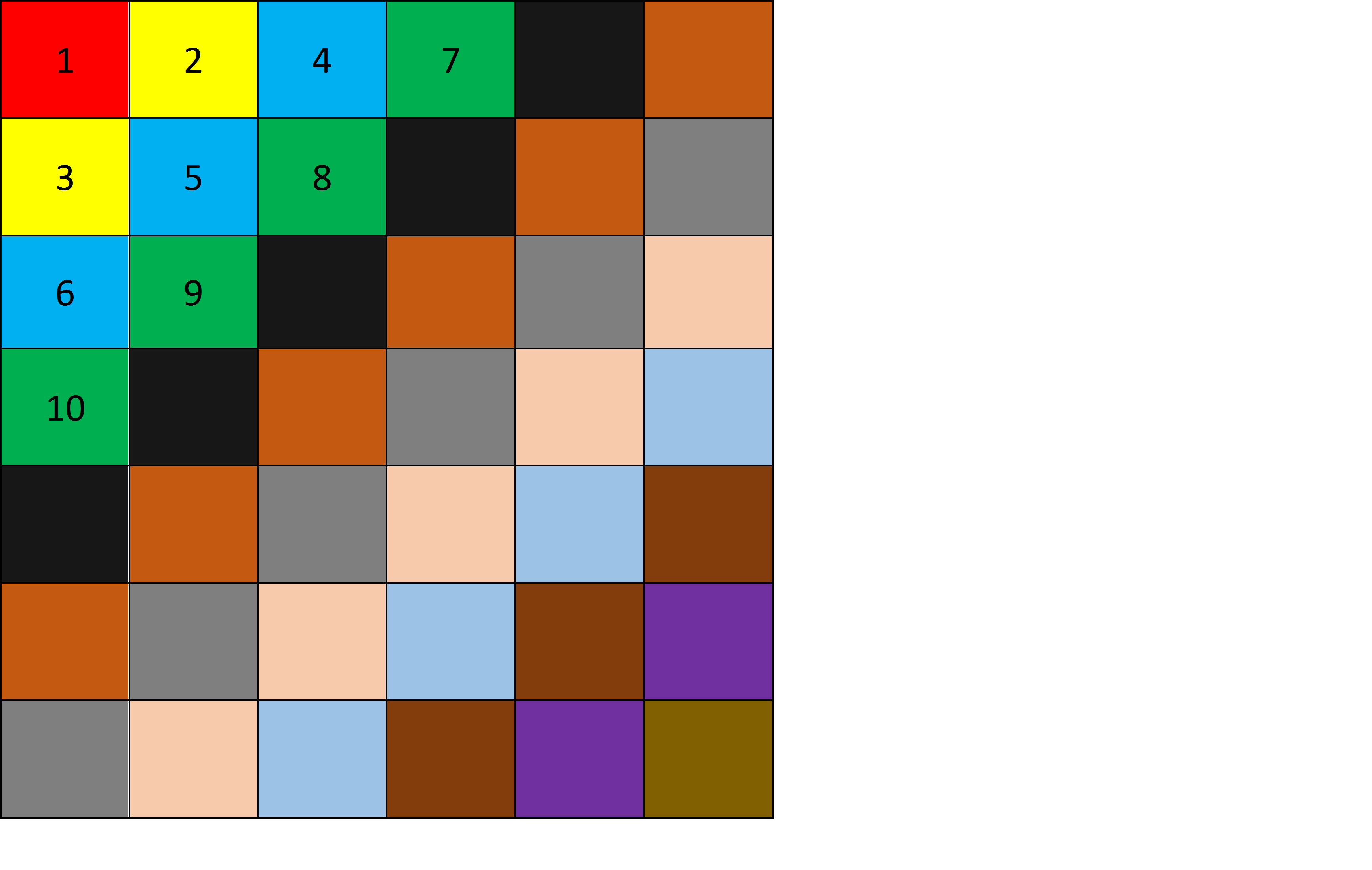# 再次迷宫救人——BFS3可直达的点有5（已遍历）和6（入队），3无用出队；

Java：

import java.util.*;

public class 迷宫救人 {

static int n;
static int m;

public static void main(String[] args) {
int flag=0;    //0表示人质为找到
int tail=0;    //指向尾结点
int maze[][]=new int[n][m];    //迷宫
int book[][]=new int[n][m];    //标志
int x[]=new int[n*m];    //队列横坐标
int y[]=new int[n*m];    //队列纵坐标
int step[]=new int[n*m];    //队列步数
int dir[][]= {
{0,1},{1,0},{0,-1},{-1,0}    //右，下，左，上
};
for(int i=0;i<n;i++) {
for(int j=0;j<m;j++) {
book[i][j]=0;
}
}
book=1;    //起始结点
//起始结点入队列
x[tail]=0;
y[tail]=0;
step[tail]=0;
tail++;    //尾指针后移
for(int i=0;i<4;i++) {
if(dx<0 || dx>n-1 || dy<0 || dy>m-1) {    //越界判断
continue;
}
if(maze[dx][dy]==1 || book[dx][dy]==1) {    //障碍点和访问与否判断
continue;
}
//符合条件，入队列
book[dx][dy]=1;
x[tail]=dx;
y[tail]=dy;
tail++;
if(maze[dx][dy]==2) {
flag=1;    //发现人质
break;
}
}
if(flag==1) {
break;
}
}